English Exercises > Other printables exercises

# Math Vocabulary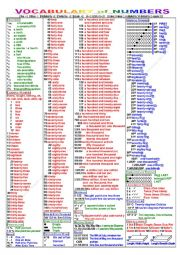NUMBERS 001 Zero to a Billion Level: elementary Age: 3-100 Downloads: 1063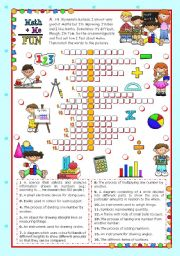Classroom objects and symbols Set (2) - Vocabulary related to Mathematics Level: intermediate Age: 9-12 Downloads: 376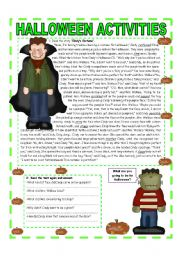OCTOBER THEME - HALLOWEEN: READING - QUESTIONS - VOCABULARY & MATH (3/3) - Lower Intermediate / Intermediate - (2 pages) Level: intermediate Age: 12-17 Downloads: 347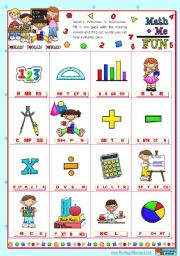Classroom objects and symbols Set (1) - Vocabulary related to Mathematics Level: elementary Age: 6-10 Downloads: 258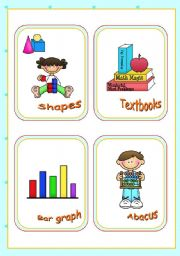Classroom objects and symbols Set (3) - Vocabulary associated with Mathematics Level: elementary Age: 6-12 Downloads: 111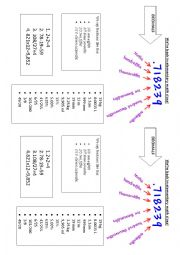Simple Math vocabulary-decimals, fractions, and measurements Level: intermediate Age: 10-17 Downloads: 47

Math Vocabulary

 Question Answer What are 2 more than 6? Ā Increase 5 by 2. Ā 5 greater than 6 is? Ā From 5 take away 2. Ā From 6 subtract 2. Ā 8 minus 3. Ā Divide 10 people in 2 equal groups. How many people in each group? Ā How many groups of three are there in 12? Ā Share 12 sweets between 4 children. How many sweets does each child get? Ā Multiply 4 by 2. Ā What is the product of 6 and 2? Ā What are 4 lots of 5? Ā What must you add to 7 to make 10? Ā You have 4 cookies how many more do you need to make 10? Ā 6 plus 8=? Ā Add 6 and 4. Ā Decrease 4 by 3. Ā Reduce 7 by 4. Ā 4 less than 6. Ā By how much is 6 less than 8? Ā Group in pairs 12 children. How many pairs of children? Ā Arrange 30 people in groups of 10. How many groups of ten are there? Ā 12 divided by 4 equals? Ā Find half of 6. Ā What are 2 times 6? Ā Double 5 equals? Ā 6 groups of 3 equal? Ā What is the sum of 2 lots of Ā4 ? Ā Add 2 to 2. Ā 3 add 4. Ā What do 3 and 7 make? Ā Find the sum of 3 and 10? Ā Which number is 4 fewer than 7? Ā What is the difference between 6 and 4? Ā How many lots of 6 are there in 18? Ā How many 3s make 21? Ā How many groups of 5 can be made out of 25? Ā How many groups of three are there in 12? Ā 3, 6 and 2 how many altogether? Ā Total 5 and 4. Ā Split 10 into 2. Ā Distribute 6 candies between 3 children. How many candies does each child get? Ā Distribute 13 candies between 2 children. What is the remainder? Ā